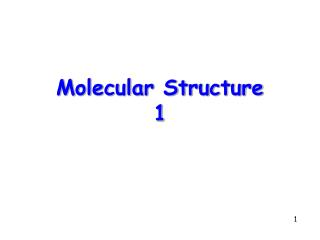DownloadDownload PresentationMolecular Structure 1

# Molecular Structure 1

Télécharger la présentation## Molecular Structure 1

- - - - - - - - - - - - - - - - - - - - - - - - - - - E N D - - - - - - - - - - - - - - - - - - - - - - - - - - -
##### Presentation Transcript

1. Molecular Structure 1

2. Topics • Introduction • The Ionic Bond • Summary

3. Introduction • Molecules are quantum systems with at least two nuclei interacting with one or more electrons • For example, the N2 molecule consists of 2 nuclei and 14 electrons • Unfortunately, it is extremely difficult to compute the properties of a molecule when viewed as an assembly of electrons and nuclei

4. Introduction • Instead, we use a much simpler (approximate) model in which a molecule is viewed as comprising atoms or ions that bond together in different ways. (An ion is a charged atom.) • The basic assumption is that bonding does not alter the identity of the atoms or ions too much. Therefore, they can be thought of as the fixed building blocks of molecules

5. Introduction Because of the Pauli exclusion principle, each electron in an atom will occupy a single quantum state, starting with those of lowest energy 4s 3p 3s 2p 2s 1s Energy

6. Introduction Example: Configuration of Nitrogen N 1s22s22p3 4s 3p 3s 2p 2s 1s Energy

7. Introduction Example: Configuration of Chlorine Cl1s22s22p63s23p5 4s 3p 3s 2p 2s 1s Energy

8. Introduction Example: Configuration of Argon Ar 1s22s22p63s23p6 4s 3p 3s 2p 2s 1s Energy

9. Introduction Example: Configuration of Potassium K 1s22s22p63s23p64s1 4s 3p 3s 2p 2s 1s Energy

10. Introduction • Atoms or ions can bond in different ways • By exchanging electrons • By sharing electrons • The two principal bonding mechanisms are called ionic and covalent bonds • The other important bonds are called dipole-dipole and metallic bonds

11. Introduction • Why do bonds occur? • The basic reason is simple: Nature likes to exist in the lowest possible energy state • Therefore, a molecule will form and be stable only if its total energy is lower than the sum of the total energies of the individual atoms when they are far apart

12. The Ionic Bond • Example: For the KCl molecule, the following must be true Energy(KCl) < Energy(K) + Energy(Cl) The total energy of the K + Cl system is taken to be zero when they are far apart • An ionic bond, typically the strongest of all bonds, is formed by exchanging electrons

13. 4s 3p 3s 2p 2s 1s Energy The Ionic Bond To create the KCl molecule we need to remove an electron from K yielding K+ and add one to Cl yielding Cl- K Cl

14. 4s 3p 3s 2p 2s 1s Energy The Ionic Bond 4.34 eV must be added to remove the outer electron 3.62 eV must be removed to add an outer electron K Cl Net energy added 4.34 – 3.62 = 0.72 eV

15. Ionization energies and electron affinities

16. 4s 3p 3s 2p 2s 1s Energy The Ionic Bond Since 0.72 eV is greater than 0 eV, it would seem that the KCl molecule would not form! K+ Cl- But…

17. The Ionic Bond …if the ions are brought within about 2.8 nm of each other K+ Cl- 2.8 nm they are pulled together and the loss in potential energy DU = ke2/r is greater than the 0.72 eV added, thereby lowering the total energy of the KCl molecule below that of the widely separated K and Cl atoms

18. Summary • It is simpler to think of molecules as composites of atoms and ions, with perhaps a few electrons, rather than as composites of nuclei and electrons • Bonding occurs if the total energy of a molecule is lower than the total energy of its constituent parts when they are far from each other • Ionic bonds are caused by the Coulomb attraction between ions